# Sådan får du en Raspberry Pi model 3 til at boote Home Assistant fra SSD disk

Det skulle være utroligt enkelt. Nettet fortæller at en Raspberry Pi 3 (og 4) per automatik kan boote fra usb-devices. Og hvis ikke den kan, så skal man bare sætte et særligt sd-kort i, der piller ved en bit i et eller andet, og så virker det.

Det gør det bare ikke, og at dømme efter de frustrerede opslag på nettet, er det ikke kun mig der har problemet.

Så skal man bare have et SD-kort med filen bootcode.bin, og så kører det, får man at vide på nettet. Det gør det også. Hver tiende gang.

Det man gør er at man placerer hele boot-partitionen på sd-kortet. Og så kører det 9 ud af 10 gange. Derefter skriver man boot_delay=2 (hvor de 2 dækker over to sekunder, det kan være det virker med 1 for dig), i config.txt. Og så virker det! Hver gang!

# ggbiplot

Bare fordi. ggbiplot er en lækker pakke. Det kan bare være en lidt frustrerende oplevelse at få den installeret. Der er bøvl med forskellige versioner af R, og når 60 studerende på et kursus alle prøver at installere fra github samtidig, støder man næsen mod begrænsninger i githubs api. Så. Kopier nedenstående, kør det i R, og funktionen ggbiplot er tilgængelig.

```ggbiplot <- function (pcobj, choices = 1:2, scale = 1, pc.biplot = TRUE,
obs.scale = 1 - scale, var.scale = scale, groups = NULL,
ellipse = FALSE, ellipse.prob = 0.68, labels = NULL, labels.size = 3,
alpha = 1, var.axes = TRUE, circle = FALSE, circle.prob = 0.69,
varname.size = 3, varname.adjust = 1.5, varname.abbrev = FALSE,
...)
{
library(ggplot2)
library(plyr)
library(scales)
library(grid)
stopifnot(length(choices) == 2)
if (inherits(pcobj, "prcomp")) {
nobs.factor <- sqrt(nrow(pcobj\$x) - 1)
d <- pcobj\$sdev
u <- sweep(pcobj\$x, 2, 1/(d * nobs.factor), FUN = "*")
v <- pcobj\$rotation
}
else if (inherits(pcobj, "princomp")) {
nobs.factor <- sqrt(pcobj\$n.obs)
d <- pcobj\$sdev
u <- sweep(pcobj\$scores, 2, 1/(d * nobs.factor), FUN = "*")
}
else if (inherits(pcobj, "PCA")) {
nobs.factor <- sqrt(nrow(pcobj\$call\$X))
d <- unlist(sqrt(pcobj\$eig))
u <- sweep(pcobj\$ind\$coord, 2, 1/(d * nobs.factor), FUN = "*")
v <- sweep(pcobj\$var\$coord, 2, sqrt(pcobj\$eig[1:ncol(pcobj\$var\$coord),
1]), FUN = "/")
}
else if (inherits(pcobj, "lda")) {
nobs.factor <- sqrt(pcobj\$N)
d <- pcobj\$svd
u <- predict(pcobj)\$x/nobs.factor
v <- pcobj\$scaling
d.total <- sum(d^2)
}
else {
stop("Expected a object of class prcomp, princomp, PCA, or lda")
}
choices <- pmin(choices, ncol(u))
df.u <- as.data.frame(sweep(u[, choices], 2, d[choices]^obs.scale,
FUN = "*"))
v <- sweep(v, 2, d^var.scale, FUN = "*")
df.v <- as.data.frame(v[, choices])
names(df.u) <- c("xvar", "yvar")
names(df.v) <- names(df.u)
if (pc.biplot) {
df.u <- df.u * nobs.factor
}
r <- sqrt(qchisq(circle.prob, df = 2)) * prod(colMeans(df.u^2))^(1/4)
v.scale <- rowSums(v^2)
df.v <- r * df.v/sqrt(max(v.scale))
if (obs.scale == 0) {
u.axis.labs <- paste("standardized PC", choices, sep = "")
}
else {
u.axis.labs <- paste("PC", choices, sep = "")
}
u.axis.labs <- paste(u.axis.labs, sprintf("(%0.1f%% explained var.)",
100 * pcobj\$sdev[choices]^2/sum(pcobj\$sdev^2)))
if (!is.null(labels)) {
df.u\$labels <- labels
}
if (!is.null(groups)) {
df.u\$groups <- groups
}
if (varname.abbrev) {
df.v\$varname <- abbreviate(rownames(v))
}
else {
df.v\$varname <- rownames(v)
}
df.v\$angle <- with(df.v, (180/pi) * atan(yvar/xvar))
df.v\$hjust = with(df.v, (1 - varname.adjust * sign(xvar))/2)
g <- ggplot(data = df.u, aes(x = xvar, y = yvar)) + xlab(u.axis.labs) +
ylab(u.axis.labs) + coord_equal()
if (var.axes) {
if (circle) {
theta <- c(seq(-pi, pi, length = 50), seq(pi, -pi,
length = 50))
circle <- data.frame(xvar = r * cos(theta), yvar = r *
sin(theta))
g <- g + geom_path(data = circle, color = muted("white"),
size = 1/2, alpha = 1/3)
}
g <- g + geom_segment(data = df.v, aes(x = 0, y = 0,
xend = xvar, yend = yvar), arrow = arrow(length = unit(1/2,
"picas")), color = muted("red"))
}
if (!is.null(df.u\$labels)) {
if (!is.null(df.u\$groups)) {
g <- g + geom_text(aes(label = labels, color = groups),
size = labels.size)
}
else {
g <- g + geom_text(aes(label = labels), size = labels.size)
}
}
else {
if (!is.null(df.u\$groups)) {
g <- g + geom_point(aes(color = groups), alpha = alpha)
}
else {
g <- g + geom_point(alpha = alpha)
}
}
if (!is.null(df.u\$groups) && ellipse) {
theta <- c(seq(-pi, pi, length = 50), seq(pi, -pi, length = 50))
circle <- cbind(cos(theta), sin(theta))
ell <- ddply(df.u, "groups", function(x) {
if (nrow(x) <= 2) {
return(NULL)
}
sigma <- var(cbind(x\$xvar, x\$yvar))
mu <- c(mean(x\$xvar), mean(x\$yvar))
ed <- sqrt(qchisq(ellipse.prob, df = 2))
data.frame(sweep(circle %*% chol(sigma) * ed, 2,
mu, FUN = "+"), groups = x\$groups)
})
names(ell)[1:2] <- c("xvar", "yvar")
g <- g + geom_path(data = ell, aes(color = groups, group = groups))
}
if (var.axes) {
g <- g + geom_text(data = df.v, aes(label = varname,
x = xvar, y = yvar, angle = angle, hjust = hjust),
color = "darkred", size = varname.size)
}
return(g)
}```
Udgivet i R

# Easter in excel

How do we calculate easter in Excel? For a spreadsheet a colleague is making for our vacation planning at work.

There are several ways. The best place I’ve found is here. A site that I’m definitely gonna study closer.

One of the formulas – translated into a danish excel-context:

`=AFRUND(DATO(A2;4;1)/7+REST(19*REST(A2;19)-7;30)*14%;0)*7-6`

# Get current wifi ssid in Python

As with a lot of the posts here. Just at quick note to make sure I can find the information again.

Print the current wlan ssid i python, running raspbian (or other linux OS’es):

```import subprocess
ssid = subprocess.check_output("iwgetid -r", shell=True)
print(ssid)```

# Themes i R

Der er sikkert bedre ovesigter. Men det her er de jeg er faldet over, og godt vil kunne huske.

TV-themes: Farver, baggrund med videre. Tematiseret efter TV-shows. Brooklyn Nine-Nine, Game of Thrones, Rick & Morty, Simpsons og Parks & Rec. Blandt mange andre.

Wes Anderson: Knap så meget temaer, som farve paletter. Der matcher Wes Andersons film.

# Project Euler 206, Concealed Square

Find the unique positive integer whose square has the form 1_2_3_4_5_6_7_8_9_0,
where each “_” is a single digit.

All right. The largest square we can get is:

``````largest <- 1929394959697989990
``````

And the smallest:

``````smallest <- 1020304050607080900
``````

That means, that the integer we are looking for is in this interval:

``````sqrt(smallest)
``````
```##  1010101010.1010101
```
``````sqrt(largest)
``````
```##  1389026623.1062636
```

We know that the square has to end on 0. That means, that the integer must also end on 0.

Any integer with 0 as the last digit, will give us a square that ends with 00.

From that we can conclude, that the square end with 900, and from that, that the integer must end with either 30 or 70.

That trims the number of integers we need to check significantly.

Although the range we are looking at, is reduced only a little:

1010101030 to 1389026670.

We are going to need a couple of libraries:

``````library(tibble)
library(dplyr)
``````
``````result <- seq(1010101030,1389026670, by = 10) %>%
enframe() %>%
filter(value%%100 %in% c(30,70)) %>%
mutate(square = (value)**2) %>%
filter(square%/%10000000000000000%%10 == 2) %>%
filter(square%/%100000000000000%%10 == 3) %>%
filter(square%/%1000000000000%%10 == 4) %>%
filter(square%/%10000000000%%10 == 5) %>%
filter(square%/%100000000%%10 == 6) %>%
filter(square%/%1000000%%10 == 7) %>%
filter(square%/%10000%%10 == 8) %>%
select(value)
``````

Not very elegant. A more detailed analysis might have given the result without having to write any code.

# Errorbars on barcharts

## Errorbars

An errorbar is a graphical indication of the standard deviation of a measurement. Or a confidence interval.

The mean of a measurement is something. We want to illustrate how confident we are of that value.

How do we plot that?

I am going to use three libraries:

``````library(ggplot2)
library(dplyr)
library(tibble)
``````

ggplot2 for plotting, dplyr to make manipulating data easier. And tibble to support a single line later.

Then, we need some data.

``````data <- data.frame(
name=letters[1:3],
mean=sample(seq(4,15),3),
sd=c(1,0.2,3)
)
``````

I now have a dataframe with three variables, name, mean and standard deviation (sd).

How do we plot that?

Simple:

``````data %>%
ggplot() +
geom_bar( aes(x=name, y=mean), stat="identity", fill="skyblue") +
geom_errorbar(aes(x=name, ymin=mean-sd, ymax = mean+sd), width=0.1)
``````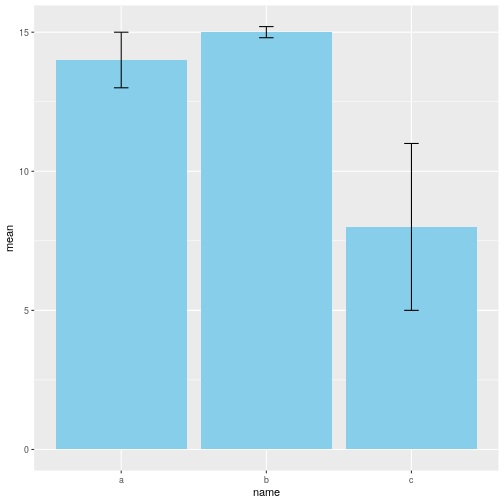So. What on earth was that?

The pipe-operator %>% takes whatever is on the left-hand-side, and inserts it as the first variable in whatever is on the right-hand-side.

What is on the right-hand-side is the ggplot function. That would normally have a datat=something as the first variable. Here that is the data we constructed earlier.

To that initial plot, which is completely empty, we add a geom_bar. That plots the bars on the plot. It takes an x-value, name, and a y-value, mean. And we tell the function, that rather than counting the number of observations of each x-value (the default behavior of geom_bar), it should use the y-values provided. We also want a nice lightblue color for the bars.

To that bar-chart, we now add errorbars. geom_errorbar needs to know the x- and y-values of the bars, in order to place them correctly. It also needs to know where to place the upper errorbar, and the lower errorbar. And we supply the information that ymin, the lower, should be the mean value minus the standard deviation. And the upper bar, ymax, the sum of the mean and sd. Finally we need to decide how broad those lines should be. We do that by writing “width=0.1”. We do not actually have to, but the default value results in an ugly plot.

And there you go, a barchart with errorbars!

## Next step

That was all very nice. However! We do not usually have a nice dataframe with means and standard deviations calculated directly. More often, we have a dataframe like this:

``````mtcars %>%
remove_rownames() %>%
select(cyl, disp) %>%
``````
```##   cyl disp
## 1   6  160
## 2   6  160
## 3   4  108
## 4   6  258
## 5   8  360
## 6   6  225
```

I’ll get back to what the code actually means later.

Here we have 32 observations (only 6 shown above), of the variables “cyl” and “disp”. I would now like to make a barplot of the mean value of disp for each of the three different values or groups in cyl (4,6 and 8). And add the errorbars.

You could scroll through all the data, sort them by cyl, manually count the number of observations in each group, add the disp, divide etc etc etc.

But there is a simpler way:

``````mtcars %>%
remove_rownames() %>%
select(cyl, disp) %>%
group_by(cyl) %>%
summarise(mean=mean(disp), sd=sd(disp))
``````
```## # A tibble: 3 x 3
##     cyl  mean    sd
##   <dbl> <dbl> <dbl>
## 1     4  105.  26.9
## 2     6  183.  41.6
## 3     8  353.  67.8
```

mtcars is a build-in dataset (cars in the us in 1974). I send that, using the pipe-operator, to the function remove_rownames, that does exactly that. We don’t need them, and they will just confuse us. That result is then send to the function select, that selects the two columns/variables cyl and disp, and discards the rest. Next, we group the data according to the value of cyl. There are three different values, 4, 6 and 8. And then we use the summarise function, to calculate the mean and the standard deviation of disp, for each of the three groups.

Now we should be ready to plot. We just send the result above to the plot function from before:

``````mtcars %>%
remove_rownames() %>%
select(cyl, disp) %>%
group_by(cyl) %>%
summarise(mean=mean(disp), sd=sd(disp)) %>%
ggplot() +
geom_bar( aes(x=cyl, y=mean), stat="identity", fill="skyblue") +
geom_errorbar(aes(x=cyl, ymin=mean-sd, ymax = mean+sd), width=0.1)
``````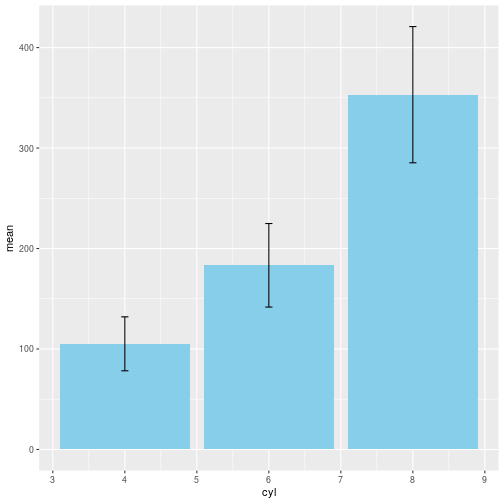All we need to remember is to change “name” in the original to “cyl”. All done!

## But wait! There is more!!

Those errorbars can be shown in more than one way.

Let us start by saving our means and sds in a dataframe:

``````data <- mtcars %>%
remove_rownames() %>%
select(cyl, disp) %>%
group_by(cyl) %>%
summarise(mean=mean(disp), sd=sd(disp))
``````

geom_crossbar results in this:

``````data %>%
ggplot() +
geom_bar( aes(x=cyl, y=mean), stat="identity", fill="skyblue") +
geom_crossbar( aes(x=cyl, y=mean, ymin=mean-sd, ymax=mean+sd))
``````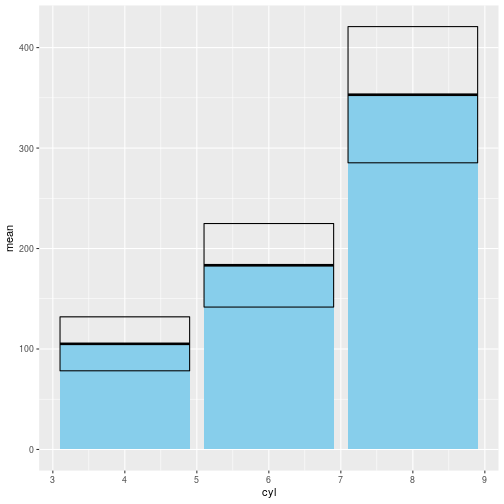I think it is ugly. But whatever floats your boat.

Then there is just a vertival bar, geom_linerange. I think it makes it a bit more difficult to compare the errorbars. On the other hand, it results in a plot that is a bit more clean:

``````data %>% ggplot() +
geom_bar( aes(x=cyl, y=mean), stat="identity", fill="skyblue") +
geom_linerange( aes(x=cyl, ymin=mean-sd, ymax=mean+sd))
``````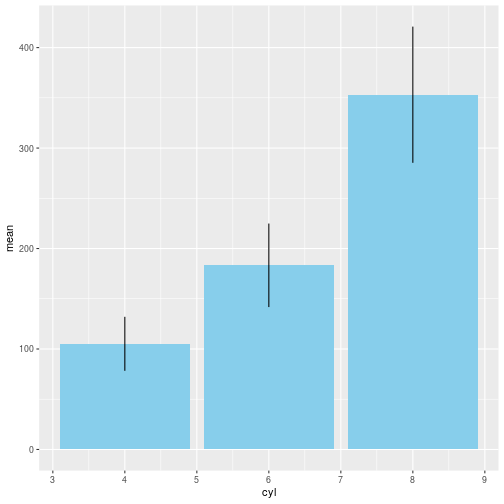And here is geom_pointrange. The mean is shown as a point. This probably works best without the bars.

``````data %>% ggplot() +
geom_bar( aes(x=cyl, y=mean), stat="identity", fill="skyblue", alpha=0.5) +
geom_pointrange( aes(x=cyl, y=mean, ymin=mean-sd, ymax=mean+sd))
``````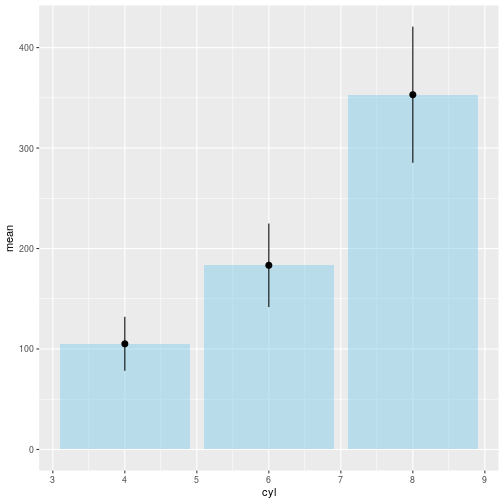# Project Euler 5 – Smallest multiple

What is the smallest, positive, number that can be divided by all numbers from 1 to 20 without any remainder?

We are given that 2520 is the smallest that can be divided by all numbers from 1:10.

One number that can definitely be divided by all numbers from 1:20 is:

``````factorial(20)
``````
```##  2.432902e+18
```

But given that

``````factorial(10)
``````
```##  3628800
```

is rather larger than 2520, it is definitely not the answer.

The answer must be a multiple of all the primes smaller than 20. A number that is divisible by 15, will be divisible by
3 and 5.

The library “numbers” have a lot of useful functions. Primes(20) returns all primes smaller than 20, and prod() returns the product of all those primes

``````library(numbers)
prod(Primes(20))
``````
```##  9699690
```

What we are looking at is the modulo-operator. 9699690 modulo 2 – what is the remainder? We know that all the remainders, dividing by 1 to 20 must be 0.

``````prod(Primes(20)) %% 2
``````
```##  0
```

And our large product is divisible by 2 without a remainder.

Thankfully the operator is vectorized, so we can do all the divisions in one go:

``````9699690 %% 1:20
``````
```##    0  0  0  2  0  0  0  2  3  0  0  6  0  0  0 10  0 12  0 10
```

Nope.

``````9699690 %% 4
``````
```##  2
```

Leaves a remainder.

``````(2*9699690) %% 4
``````
```##  0
```

Now I just need to find the number to multiply 9699690 with, in order for all the divisions to have a remainder of 0.
That is, change i in this code until the answer is true.

``````i <- 2
all((i*9699690) %% 1:20 == 0)
``````
```##  FALSE
```

Starting with 1*9699690, I test if all the remainders of the divisions by all numbers from 1 to 20 is zero.
As long as they are not, I increase i by 1, save i*9699690 as the answer, and test again.
If the test is TRUE, that is all the remainders are 0, the while-loop quits, and I have the answer.

``````i <- 1
while(!all((i*9699690) %% 1:20 == 0)){
i <- i + 1
}
``````

# Project Euler 4 – Largest palindrome product

I solved this more than four years ago. So I have no idea if this was the way I did it originally.

The problem is:

A palindromic number is a number that reads the same both ways. Like “A man, a plan, a canal, Panama!” just with numbers.

The problem informs us, that the largest palindrome made from the product of two two-digit numbers, is 9009.

We get that from 91 × 99. We must now find the largest palindrome, made from the product of two three-digit numbers.

First, we need to figure out how to test if a number is palindromic. There might be easier ways. But this is mine.

Convert the number to a string. Split it in individual characters reverse the string, and compare to the original.

Like this, using the stringr library:

``````library(stringr)
rev(unlist(str_split("9009", ""))) == unlist(str_split("9009", ""))
``````
```##  TRUE TRUE TRUE TRUE
```

Everything is TRUE, as it should be – lets make a function.

``````is_palindromic <- function(x){
all(rev(unlist(str_split(x, ""))) == unlist(str_split(x, "")))
}

is_palindromic(9009)
``````
```##  TRUE
```

Only problem is that it is not vectorized

``````is_palindromic(c(991, 9009))
``````
```##  FALSE
```

Just for the fun of it, lets write something vectorized. This is definitely not the way I did it originally.

``````is_palindromic <- function(x){
unlist(lapply(lapply(str_split(x,""), rev),str_c, collapse="")) == as.character(x)
}

is_palindromic(c(991, 9009))
``````
```##  FALSE  TRUE
```

Nice.
There are definitely faster ways of doing it.

Now I just need to generate all products made up of two three-digit numbers, test if it is palindromic, and find the largest.

I use expand.grid to generate all combinations of all three-digit numbers, transmute the product into existence, and filter out all the non-palindromic products. Then I arrange the product variable in descending order, and take the first.

``````library(dplyr)
transmute(product = Var1*Var2) %>%
filter(is_palindromic(product)) %>%
arrange(desc(product)) %>%
slice(1)
``````

Not very fast… The main problem is that I check all 810.000 products. There is no need for that. If I instead test the products in order, from the largest, to the smallest, the first palindromic number I find, is the largest.

Let us generate a list of candidates, in descending order:

``````cand <- expand.grid(100:999, 100:999) %>%
transmute(product = Var1*Var2) %>%
arrange(desc(product)) %>%
.\$product
``````

And now, for each element in the candidate-vector, if it is palindromic, set that to be the answer, and break out of the for-loop.

``````for(i in cand){
if(is_palindromic(i)){
break()
}
}
``````

Much faster.

# Transfer a file between two computers using SSH

I have a Raspberry Pi Zero w, have connected to it using SSH from a laptop running Ubuntu. Now I want to transfer a file from the laptop, to the Raspberry Pi.

sudo rsync /path/to/local/file pi@raspberrypi.local:/home/pi

Simple